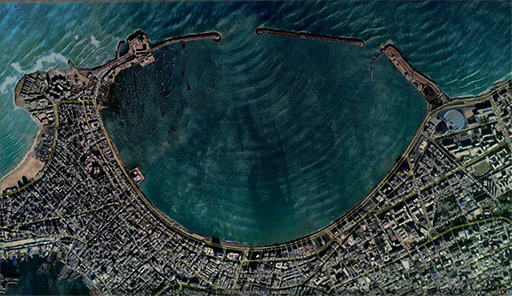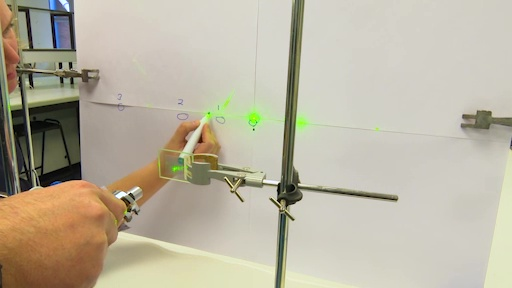Microgravity: living on the International Space Station

Start this free course now. Just create an account and sign in. Enrol and complete the course for a free statement of participation or digital badge if available.

Free course

# 3 Diffraction of waves

Before you journey into the quantum world, you need to consider some physical effects that can be seen in your everyday life. Diffraction of waves is one of them. For example, when the entrance to a harbour is of the right size, water waves diffract, or spread out, as they move into the harbour (Figure 4).Figure 4 Water wave diffraction in a harbour.

If you click here [Tip: hold Ctrl and click a link to open it in a new tab. (Hide tip)] , it will take you to an online animation where you can change the gap size for single slit and ripple tank simulations. Note carefully what happens to the waves, then try Activity 2.

## Activity 2 Exploring diffraction

Timing: Allow approximately 15 minutes

Choose the one correct option to complete the following statements based on the animation.

Active content not displayed. This content requires JavaScript to be enabled.
Interactive feature not available in single page view (see it in standard view).
Active content not displayed. This content requires JavaScript to be enabled.
Interactive feature not available in single page view (see it in standard view).
Active content not displayed. This content requires JavaScript to be enabled.
Interactive feature not available in single page view (see it in standard view).

You can also see diffraction effects using LASER light. Watch Video 2 where this effect is demonstrated using LASER pens and diffraction gratings. Then complete Activity 3.Skip transcript: Video 2 LASER pen diffraction experiment.

#### Transcript: Video 2 LASER pen diffraction experiment.

TOM:
Welcome to next practical we're going to do, which is based on the laser pen of different wavelengths and also some diffraction gratings as well. The first diffraction grating we're going to look at is of order 600 lines per millimetre. Then we're going to look at one which has 300 lines per millimetre.
What we're going to do is show that light can behave like a wave as well as a particle. And for that we need, this important equation up on the board here, n lambda equals d sin theta. We'll be looking at what we changed is how it's going to affect the outcome. So we'll have a wave length and an angle here as well.
For the first part of the practical, we've got a blue laser, and we're going to put it through a diffraction grating here of 600 lines per millimetre. And the blue laser, as it goes through, has spots that come up to the centre, to the left, and to the right. Helen will mark these spots as we go through and see the one in the centre and three to the left.
Now number them 1, 2, and 3. That's the order from the centre is naught, 1, 2, and 3 to the left. That's from the blue laser.
Now what would happen if we changed the colour of the laser? We're now going to go for different colour laser. And again, we're going to use the same diffraction grating, and we're putting a green light through the same diffraction grating.
Now you'll see with a different colour, the spots are in different locations. The one at number 1, number 2 have moved slightly more.
Now we'll change the colour of the laser. Now let's try red coloured laser going through the centre of the 300 lines per millimetre diffraction grating. You see it's quite hard to see the next spot with the red laser.
Now we've used different laser pens with the same diffraction grating. We're now going to change the diffraction grating from 600 lines per millimetre to 300 lines millimetre.
I will start back with the blue coloured laser, I'm going to move that one out of the way. And we'll move. We're now with the blue laser going into diffraction grating of 300 lines per millimetre.
Now you see with the locations of the blue spots in the middle, and the blue spot compared before has now moved considerably to the right, the closer to the centre. So now the numbers being marked up are number 1, number 2 are closer to a centre. Do we expect that to happen for the other coloured lasers? Let's have a look.
You remember last time on the 600 line millimetre, we had number 1 to the left, and again, we've got number 1 to the left, which is much closer to the centre than before. Now we have number 1, number 2, number 3, number 4, and even number 5.
And finally, for the last laser, again, we can make our expectations of what's going to happen. And we'll try that with the same, with a red laser, and we'll see again the spots have moved to the left in comparison with number 1 before.
The conclusion of what we've done here is we look through the material in the course as we're working through. And the conclusion is the laser going through diffraction gratings of different number of lines per millimetre is affected by the equation n lambda equals d sin theta. If you change one thing, you will change the outcome of your experiment.
End transcript: Video 2 LASER pen diffraction experiment.
Video 2 LASER pen diffraction experiment.
Interactive feature not available in single page view (see it in standard view).

Here, the concept of light behaving as a particle and a wave is introduced. Later on, you will see how electrons, as small particles of matter, can also behave as particles and waves.

## Activity 3 The LASER diffraction experiment

Timing: Allow approximately 15 minutes

Choose the one correct answer from the options given to complete the following statements.

1. Moving out from the centre of the board, the order of the colours was as follows.

a.

red and green

b.

blue, green and red

c.

yellow and red

d.

green and blue

e.

green, red, blue and yellow

2. The diffraction gratings used, in terms of lines per millimetre (mm), were:

a.

300

b.

1200

c.

600 and 1200

d.

300 and 600

e.

300

3. As the number of lines per mm on the diffraction gratings decreased, the spots on the board:

a.

moved further away

b.

moved closer

c.

were unchanged

As the number of lines per millimetre on the diffraction grating decreases, the distance d between the lines increases. When this happens, the angle θ increases which affects the distances between the spots in Question 3 above. But how are these terms related to each other? Video 2 introduced Equation 1 which can now be explained in more detail.

Equation label: Equation 1

n is an integer (whole number) as you count the dots from the centre outwards; the central dot is labelled n = 0.

λ is the wavelength of the LASER and is measured in m.

d is the separation between adjacent lines on the diffraction grating, again measured in m.

θ is the angle of diffraction from the LASER pen to the individual dots.

sin(θ) is the sine of the angle θ; this can be calculated by using the ‘sin’ button on your calculator.

You will now look at sodium D-lines and the simplest atom of all, hydrogen.

MG_1